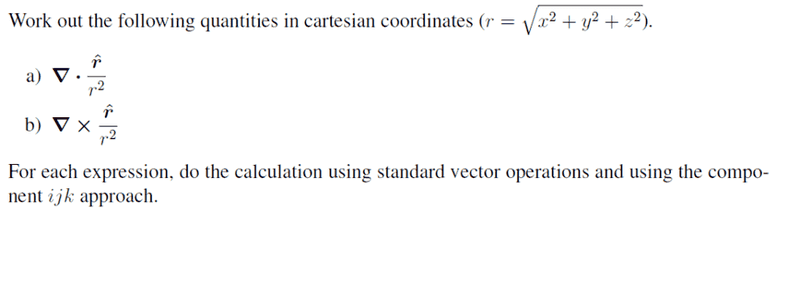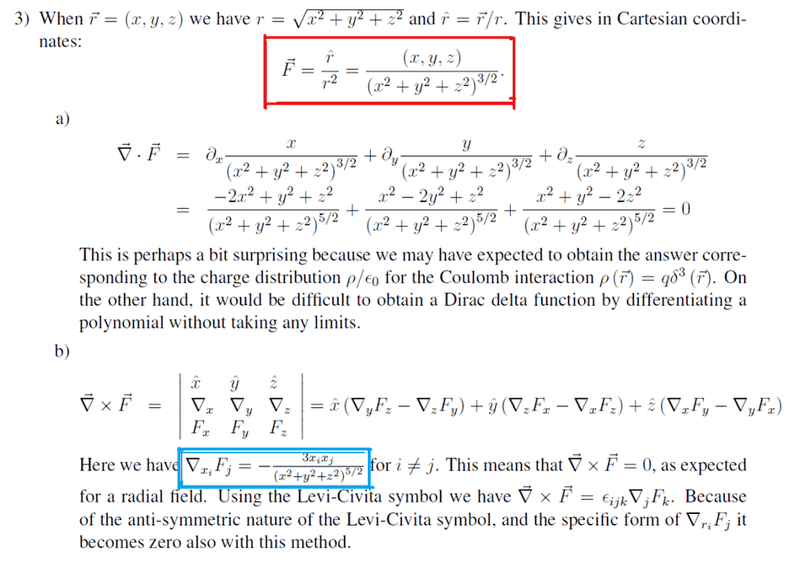# I really don't know what this is ><

quietrain

## Homework Statement## Homework Equations## The Attempt at a Solution

can anyone enlighten me what the red and blue equations mean? i remembered something about the red eqn meaning unit vector in vector format, or scalar format is it?

and the blue eqn i have totally no idea what my prof is doing >< is he trying to say that del F is a dot product and since they are 0 because i =/= j, that means that cross product is 0???

help appreciated!

Last edited:

Homework Helper
hi quietrain !it's all very simple …

r^/r2 = (r/|r|)/r2 = r/r3

and

∂/∂xi (Fj)

= ∂/∂xi (xj)

= ∂/∂xi (xj/r3)

= {∂/∂xi (xj)}/r3 + {∂/∂xi 1/r3)}xj (product rule)

= 0 + {∂/∂xi 1/r3)}xjquietrain
with regards to part b)

for the cross product (del X F) to be 0, are they saying that delyFz - delzFy must be 0 and the other permutations too,

so that delyFz = delzFy

but from the form ∂/∂xi (Fj), it has a minus sign after differientiating, thus for Eijk, if we swop once to Eikj , then the minus sign is gone

so now its minus - plus = 2 minus , not 0?

Homework Helper
hi quietrain!(have a del: ∇ and an epsilon: ε)
for the cross product (del X F) to be 0, are they saying that delyFz - delzFy must be 0 and the other permutations too,

so that delyFz = delzFy

yesbut from the form ∂/∂xi (Fj), it has a minus sign after differientiating, thus for Eijk, if we swop once to Eikj , then the minus sign is gone

so now its minus - plus = 2 minus , not 0?

ah, you can either write ∇jFk - ∇kFj

or εijkjFk

same thingquietrain
hi quietrain!(have a del: ∇ and an epsilon: ε)

yesah, you can either write ∇jFk - ∇kFj

or εijkjFk

same thingoh so it becomes minus minus minus which is minus plus = 0
thanks!